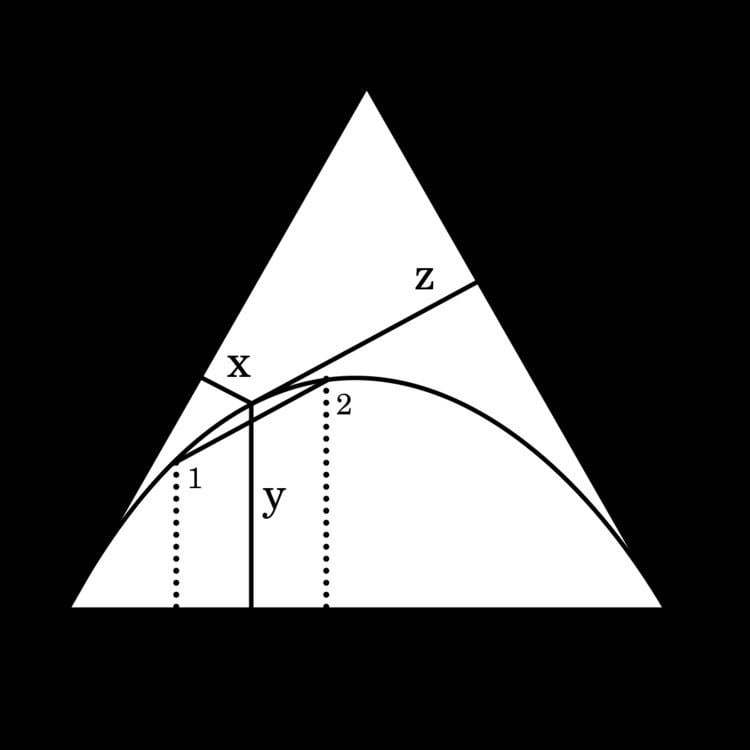# Genotype frequency

Updated on
Edit
Like
CommentGenetic variation in populations can be analyzed and quantified by the frequency of alleles. Two fundamental calculations are central to population genetics: allele frequencies and genotype frequencies. Genotype frequency in a population is the number of individuals with a given genotype divided by the total number of individuals in the population. In population genetics, the genotype frequency is the frequency or proportion (i.e., 0 < f < 1) of genotypes in a population.

Although allele and genotype frequencies are related, it is important to clearly distinguish them.

Genotype frequency may also be used in the future (for "genomic profiling") to predict someone's having a disease or even a birth defect. It can also be used to determine ethnic diversity.

## Numerical example

As an example, let's consider a population of 100 four-o-'clock plants (Mirabilis jalapa) with the following genotypes:

• 49 red-flowered plants with the genotype AA
• 42 pink-flowered plants with genotype Aa
• 9 white-flowered plants with genotype aa
• When calculating an allele frequency for a diploid species, remember that homozygous individuals have two copies of an allele, whereas heterozygotes have only one. In our example, each of the 42 pink-flowered heterozygotes has one copy of the a allele, and each of the 9 white-flowered homozygotes has two copies. Therefore, the allele frequency for a (the white color allele) equals

f ( a ) = ( A a ) + 2 × ( a a ) 2 × ( A A ) + 2 × ( A a ) + 2 × ( a a ) = 42 + 2 × 9 2 × 49 + 2 × 42 + 2 × 9 = 60 200 = 0.3

This result tells us that the allele frequency of a is 0.3. In other words, 30% of the alleles for this gene in the population are the a allele.

Compare genotype frequency: let's now calculate the genotype frequency of aa homozygotes (white-flowered plants).

f ( a a ) = 9 49 + 42 + 9 = 9 100 = 0.09 = ( 9 % )

Allele and genotype frequencies always sum to less than or equal to one (in other words, less than or equal to 100%).

The Hardy–Weinberg law describes the relationship between allele and genotype frequencies when a population is not evolving. Let's examine the Hardy–Weinberg equation using the population of four-o'clock plants that we considered above:
if the allele A frequency is denoted by the symbol p and the allele a frequency denoted by q, then p+q=1. For example, if p=0.7, then q must be 0.3. In other words, if the allele frequency of A equals 70%, the remaining 30% of the alleles must be a, because together they equal 100%.

For a gene that exists in two alleles, the Hardy–Weinberg equation states that (p2) + (2pq) + (q2) = 1
If we apply this equation to our flower color gene, then

f ( A A ) = p 2 (genotype frequency of homozygotes) f ( A a ) = 2 p q (genotype frequency of heterozygotes) f ( a a ) = q 2 (genotype frequency of homozygotes)

If p=0.7 and q=0.3, then

f ( A A ) = p 2 = (0.7)2 = 0.49 f ( A a ) = 2 p q = 2×(0.7)×(0.3) = 0.42 f ( a a ) = q 2 = (0.3)2 = 0.09

This result tells us that, if the allele frequency of A is 70% and the allele frequency of a is 30%, the expected genotype frequency of AA is 49%, Aa is 42%, and aa is 9%.

Genotype frequencies may be represented by a De Finetti diagram.

Genotype frequency Wikipedia